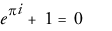informalequation

informalequation — A displayed mathematical equation without a title.

Synopsis

informalequation ::=

Attributes

• floatstyle
• pgwide (enumeration)
• “0”
• “1”
• type

Description

An informalequation is usually a mathematical equation or a group of related mathematical equations.

Processing expectations

Formatted as a displayed block.

Attributes

floatstyle

Specifies style information to be used when rendering the float

pgwide

Indicates if the element is rendered across the column or the page

type

Identifies the type of equation

Parents

These elements contain informalequation: .

Children

The following elements occur in informalequation: alt, caption, info (db.titleforbidden.info), mathphrase, mediaobject, mml:*.

Related elements: .

Examples

1<article xmlns='http://docbook.org/ns/docbook'>
<title>Example informalequation</title>

<para>The equation
5<informalequation>
<alt>e^(pi*i) + 1 = 0</alt>
<mediaobject>
<imageobject condition="print">
<imagedata fileref="figs/print/db5d_refeqn02.pdf"/>
10    </imageobject>
<imageobject condition="web">
<imagedata fileref="figs/web/db5d_refeqn02.png"/>
</imageobject>
</mediaobject>
15</informalequation>
is delightful because it joins together five of the most
important mathematical constants.
</para>

20</article>

The equationis delightful because it joins together five of the most important mathematical constants.